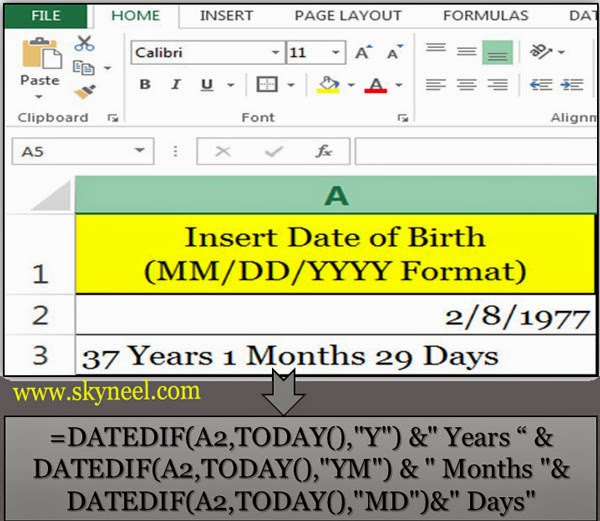# Calculating Person’s current age using DATEDIF in Excel

Use tips to quickly find your current age using DATEDIF function from the birth date till current date in Excel.

Suddenly, if anyone ask a question to you “What is your age”? Or “How old are you”?, you are not in this condition to give quick reply of this question and try to make calculation on your finger or take paper and pen and calculate your current age, but this is not the right way to known your current age, so Microsoft Excel provide us a function from “Date and Time” category, to quick calculate current age using DATEDIF function from your date of birth.

If you want to know current age of your all family members and your best friends then you have to create a list in Microsoft Excel, which keep track of all information related of your date of birth and age by using “DATEDIF” function.

How to calculate today’s your current age with the help of most popular DATEDIF ( ) Function in MS Excel. Excel’s DATEDIF function used to calculate any person’s present age in number of years, month and days. The DATEDIF function is used to calculate the difference between given two dates and print no. of years, no. of months and no. of days from given two dates.

The syntax for DATEDIF is as follows:=DATEDIF(Date1, Date2, Interval) Where:

Date1 is the Primary Date, Date2 is the Secondary Date and Interval is the type of interval which you want to return. But during typing this formula if you make any kind of mistake it displays an error message on the screen which is started by hash (#) symbol.

There are some interval values are given below with the meaning and description:

Interval Meaning Description
m Displays the number of completed calendar months.
d Displays the number of completed calendar days.
Y Displays the number of completed calendar years.
ym Displays the number of completed calendar months excluding years
yd Display the number of completed calendar days excluding years.
md Displays the number of completed days excluding years and months

Note: During typing the formula in excel sheet, if you don’t want to enter value of interval, MS Excel dispels an error message on the screen #NUM.

## How to calculate current age using DATEDIF:

=DATEDIF (A2, TODAY( ),”Y”) & ” Years ” & DATEDIF (A2, TODAY( ),”YM”) & ” Months ” & DATEDIF (A2, TODAY( ),”MD”) & ” Days”You can also distribute this formula in three parts:

#### To Calculate Number of Years:

=DATEDIF(A2,TODAY(), “y”) & ” Years”

#### To Calculate Number of Months:

=DATEDIF(A2,TODAY(), “ym”) & ” Months”

#### To Calculate Number of Days:

=DATEDIF(A2,TODAY(), “MD”) & ” Days”

#### Concatenating the Formula Together

The ampersand operator (&) is used to combine concatenation or join different data in a single formula. For example, The (&) operator is used with the DATEDIF function to join the different part of formula.

#### The TODAY( ) Function

It is a  most popular function which is used to display current date in active cell. You can combine this formula in this post with the DATEDIF formula.

I hope you like this current age using DATEDIF guide from which you will be able to easily calculate current age using DATEDIF without any extra efforts. If you feel any problem in this guide then please use comment box.